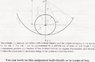# Rolling cylinder on cylindrical plane

• maffra

See attached

## Homework Equations

K.E (rotational) = .5*I*omega^2
K.E (translational) = .5*m*v^2

## The Attempt at a Solution

v(of rod and translational vel of cylinder) = L * (dαlpha/dt)

Is the angular velocity of the cylinder the same as the angular velocity of the rod?
To calculate the translational K.E of the cylinder can I use the vel I've calculated above for the rod?
For the rotational vel of the cylinder, can i use I (com) = 0.5*m*r^2

#### Attachments

•Question 3.jpg
25.2 KB · Views: 335
Is the angular velocity of the cylinder the same as the angular velocity of the rod?

The angular velocity of the CM of the cylinder about O will be equal to the angular velocity of the rod about O. But the cylinder has a different angular velocity wrt its CM.

To calculate the translational K.E of the cylinder can I use the vel I've calculated above for the rod?

Yes.

For the rotational vel of the cylinder, can i use I (com) = 0.5*m*r^2

Yes. But to find the MI about O, you have use parallel axis theorem.